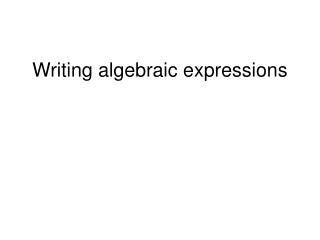Download PresentationWriting algebraic expressions

# Writing algebraic expressions - PowerPoint PPT PresentationDownload Presentation## Writing algebraic expressions

- - - - - - - - - - - - - - - - - - - - - - - - - - - E N D - - - - - - - - - - - - - - - - - - - - - - - - - - -
##### Presentation Transcript

1. Writing algebraic expressions

2. Problem: Ms. Jensen likes to divide her class into groups of 2. Use mathematical symbols to represent all the students in her class.

3. Problem: Ms. Jensen likes to divide her class into groups of 2. Use mathematical symbols to represent all the students in her class. Solution: Let g represent the number of groups in Ms. Jensen's class. Then 2 · g, or 2g can represent "g groups of 2 students".

4. Write each phrase as an algebraic expression Phrase The sum of nine and eight The sum of nine and a number

5. Write each phrase as an algebraic expression Phrase Expression The sum of nine and eight 9+8 The sum of nine and a number 9+x

6. Write each phrase as an algebraic expression Phrase Nine increased by a number x Fourteen decreased by a number p Seven less than a number t The product of 9 and number n Thirty-two divided by a number y

7. Write each phrase as an algebraic expression Phrase Expression Nine increased by a number x 9 + x Fourteen decreased by a number p 14 - p Seven less than a number t t - 7 The product of 9 and number n 9n Thirty-two divided by a number y 32/y

8. A small company has \$1000 to distribute to its employees as a bonus. How much money will each employee get? Let e represent the number of employees in the company. The amount of money each employee will get is represented by the following algebraic expression: 1000/e

9. An electrician charges \$45 per hour and spends \$20 a day on gasoline. Write an algebraic expression to represent his earnings for one day. • Let x represent the number of hours the electrician works in one day. The electrician's earnings can be represented by the following algebraic expression: 45x - 20

10. 1. Fifteen less than twice a number A. 15 - 2xB. 2x + 15C. 2x - 15D. None of the above.

11. 1. Fifteen less than twice a number A. 15 - 2xB. 2x + 15C. 2x - 15D. None of the above.

12. 2. Three times a number, increased by seventeen • 3(a + 17) • 3a + 17 • (17 + 3)a • None of the above.

13. 2. Three times a number, increased by seventeen • 3(a + 17) • 3a + 17 • (17 + 3)a • None of the above.

14. 3. The product of nine and a number, decreased by six • 9(m - 6) • 6(9 - m) • 9m – 6 • None of the above.

15. 3. The product of nine and a number, decreased by six • 9(m - 6) • 6(9 - m) • 9m – 6 • None of the above.

16. 4. Thirty divided by seven times a number • 30 + 7n • 30(7n) • 7n/30 • None of the above.

17. 4. Thirty divided by seven times a number • 30 + 7n • 30(7n) • 7n/30 • None of the above.

18. 5. Jenny earns \$30 a day working part time at a supermarket. Write an algebraic expression to represent the amount of money she will earn in d days. • 30d • 30 + d • 30/d • None of the above

19. 5. Jenny earns \$30 a day working part time at a supermarket. Write an algebraic expression to represent the amount of money she will earn in d days. • 30d • 30 + d • 30/d • None of the above